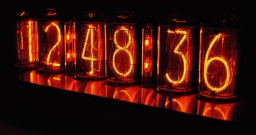# Eight years ago

The son asks his father how old he is. My father said: I will have twice as much as I had eight years ago in ten years.

x =  26

### Step-by-step explanation:

x+10 = 2·(x-8)

x = 26

x = 26/1 = 26

x = 26

Our simple equation calculator calculates it.Did you find an error or inaccuracy? Feel free to write us. Thank you!

Tips for related online calculators
Do you have a linear equation or system of equations and looking for its solution? Or do you have a quadratic equation?
Do you want to convert time units like minutes to seconds?